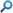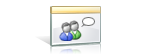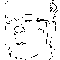# MathsShare

 This Site This ListMathsShare > Puzzles > Pegs

## Puzzles### Quick Launch View All Site ContentView: FlatPosted ByPost
 Started: 08/09/2010 03:49 PM View PropertiesJohn RowlandPegs
Which is the best fit? A square peg in a round hole or a round peg in a square hole.Posted: 21/05/2013 04:15 PM View PropertiesJohn RowlandSquare peg in round hole of radius r. Cross Section Analysis: Area of Hole = (PI)rr, Length of side of peg = 2rCos45 = 2r/sqrt(2), Area of Peg = 2rr, Peg area % = 2/(PI) = 64%

Round peg of radius r in square hole. Cross Section Analysis: Area of Hole = 4rr, Area of Peg = (PI)rr, Peg area % = (PI)/4 = 79%

(rr = r squared)

In general terms a round peg in a square hole occupies more of the area of the hole than a square peg in a round hole.